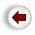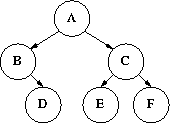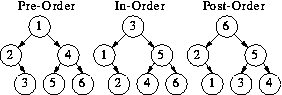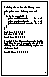# 2. Tree TraversalWhat I've just called ``scanning through'' a tree is actually called traversing a tree.

General Definition: to traverse a data structure is to process, however you like, every node in the data structure exactly once.

Note: You may ``pass through'' a node as many times as you like but you must only process the node once.
E.g. we can talk about ``traversing a list'', which means going through the list and processing every node once. We had a special name for this: `map`.

For a specific data structure, we talk about the different orders in which it might be traversed. For a list there are two common traversal orders: first-to-last (the most common) and last-to-first.

The general recursive pattern for traversing a (non-empty) binary tree is this: At node N you must do these three things:

• (L) recursively traverse its left subtree. When this step is finished you are back at N again.

• (R) recursively traverse its right subtree. When this step is finished you are back at N again.

• (N) Actually process N itself.
We may do these things in any order and still have a legitimate traversal. If we do (L) before (R), we call it left-to-right traversal, otherwise we call it right-to-left traversal.
Pre-Order Traversal
do (N) before anything else.
Post-Order Traversal
do (N) after everything else.
In-Order, or Infix Order, Traversal
do (N) in between the two subtrees.
E.g. let us look at what order the nodes will get processed given this tree:
``
Using Left-To-Right traversal:
``For example, suppose we were writing out the nodes of the tree:

```        void print_tree(binary_tree *t)
{
if (! is_empty(t)) {
print_tree(t->left);     /* L */
print_tree(t->right);    /* R */
printf("%d\n",t->value); /* N */
}
}```
The preceding diagrams show us the order in which the nodes will get written:
• Pre-Order: A-B-D-C-E-F
• In-Order: B-D-A-E-C-F
• Post-Order: D-B-E-F-C-A